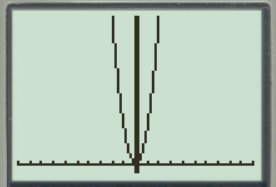# To graph : The function y = 100 x 2 in a graphing window.### Precalculus: Mathematics for Calcu...

6th Edition
Stewart + 5 others
Publisher: Cengage Learning
ISBN: 9780840068071### Precalculus: Mathematics for Calcu...

6th Edition
Stewart + 5 others
Publisher: Cengage Learning
ISBN: 9780840068071

#### Solutions

Chapter 1.9, Problem 11E
To determine

## To graph: The function y=100x2 in a graphing window.

Expert Solution

### Explanation of Solution

Given information:

The equation of the function y=100x2 .

Graph:

The graph of the function y=100x2 .

Substitute x=0 , in the equation y=100x2 ,

y=100x2y=0

Substitute x=1 , in the equation y=100x2 ,

y=100x2y=100(1)2y=100

Substitute x=2 , in the equation y=100x2 ,

y=100x2y=100(2)2y=100(4)y=400

Observe that as the value of x increases there is the huge increment in value of y .

Steps to plot the graph of the equation y=100x2 with the help of graphing utility are as follows:

Step 1: Press MODE key.

Step 2: Use the down arrow key to reach FUNC option.

Step 3: Press ENTER key.

Step 4: Press Y= key.

Step 5: Enter the function 100x2 .

Step 6: Press WINDOW key. Change the settings to

Xmin=10Xmax=10Ymin=20Ymax=400

For better view of graph.

Step 8: Press GRAPH key.

The result obtained on the screen is provided below,Interpretation:

The equation of the function y=100x2 represents a parabola.

The parabola opens upward.

The x intercepts are the points on xaxis where the graph of the equation touches xaxis .

Substitute y=0 in the equation y=100x2 ,

0=100x2x2=0100x2=0x=0

Therefore, x- intercept is 0.

The y- intercepts are the points on x -axis where the graph of the equation intersects the y -axis.

Substitute x=0 in the equation y=100x2 ,

y=100x2y=100(0)2y=1000y=0

Therefore, y- intercept are is 0.

Therefore, the equation y=100x2 is not symmetric about the x -axis.

Recall that the function is symmetric with respect to the y -axis, when x is replaced by -x .

Replace x by x in the equation y=100x2 ,

y=100(x)2y=100x2y=100x2

Therefore, the equation y=100x2 is symmetric about the y axis Initially the graph of the function y=100x2 increases when increase the value of x ,the graph is a parabola shaped graph.

Therefore, the function is open upward with axis as y -axis.

### Have a homework question?

Subscribe to bartleby learn! Ask subject matter experts 30 homework questions each month. Plus, you’ll have access to millions of step-by-step textbook answers!